Username Password Are you new? Sign up now I’ve forgotten Home :. Downloads :. Articles :. Projects :. Reviews :. Forums :. Arcade :. Klikcast :. GOTW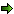Post ReplyPost Oekaki

 Posted By MessageSketchyCornwall UKRegistered  06/11/2004 Points  1960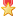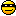15th July, 2009 at 22:52:13 - I've been trying for the last day or two, to figure out vectors. Wikipedia just left me completely baffled, with all it's dot products, and cross products, and unit vectors etc. Anyway, to try and make it a bit more "MMF2-friendly" I made a simple widget to perform some basic functions on 2d vectors. Just incase it might be useful to someone else, here it is: http://cid-b1e7ee094271bbda.skydrive.live.com/self.aspx/Public/My%20Vector%20Widget.mfa Also, I will post the specific problem I've been having, incase someone here does actually understand vectors. Just need to draw a diagram first...   n/aSketchyCornwall UKRegistered  06/11/2004 Points  196015th July, 2009 at 23:16:41 - okay, here's my problem:I know the X and Y components of vector A (the red line) - I know how to find it's length and angle too, if that helps. I know angle A (the angle of the green line from 0 degrees, ie. right) I need to find the vector (blue line) which is perpendicular to the green line, and which goes to the end of vector A. I then need to find the point 25% of the way along it. I then need to find the vector from the start of vector A, to that point. IMPORTANTLY, it needs to work for *any* angle, and *any* vector. It needs to work in all 4 quadrants not just the one shown. I think this is why I couldn't make it work using trig.   n/aCecilectomynoPERegistered  19/03/2005 Points  305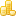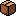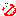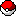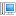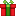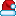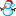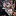15th July, 2009 at 23:19:42 - http://blog.wolfire.com/2009/07/linear-algebra-for-game-developers-part-1/ http://blog.wolfire.com/2009/07/linear-algebra-for-game-developers-part-2/ http://www.ies.co.jp/math/products/vector/menu.html http://chortle.ccsu.edu/VectorLessons/vectorIndex.html   n/aSketchyCornwall UKRegistered  06/11/2004 Points  196016th July, 2009 at 01:12:06 - Thanks for the links. I have read *a lot* about it so I have the formulae now - it's just trying to figure out how to apply them to my problem. Actually, I discovered what I really need is a little more complicated than I said before, so you can forget that until I work out exactly what I do need.   n/aDMT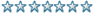Registered  18/06/2009 Points  150 16th July, 2009 at 02:14:39 - Or um... pythagorean theorem?   I am no longer not a taco.[DELETED]Likes to put dots on paperRegistered  08/12/2008 Points  118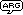16th July, 2009 at 02:38:15 - Originally Posted by DMTOr um... pythagorean theorem? Yes I believe with the formula re-arranged to some extent, you should be able to find any side of the triangle, given the other two sides.   n/aDMTRegistered  18/06/2009 Points  150 16th July, 2009 at 02:52:11 - or trignometry!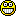(all posts must be at least 4 words long)   I am no longer not a taco.Muz Registered  14/02/2002 Points  6499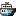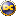16th July, 2009 at 12:59:18 - atan2 works in all quadrants, unlike atan.   Disclaimer: Any sarcasm in my posts will not be mentioned as that would ruin the purpose. It is assumed that the reader is intelligent enough to tell the difference between what is sarcasm and what is not.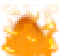DeadmanDinesBest Article WriterRegistered  27/04/2006 Points  4758 17th July, 2009 at 00:20:06 - This is pure theory, but... The sum of the 3 angles inside a triangle must be 180 degrees. So, you know ANGLE A (from baseline to green line), and you know the angle of VECTOR A (from baseline to vector A). We'll call this latter one ANGLE B to avoid confusion. Subtracting Angle A from Angle B will give you the bottom left angle within the triangle (the angle where the RED and GREEN lines meet). We already know that the blue line we are trying to plot must be at 90 degrees of the GREEN line, so the remainder is the angle where the red line meets the blue line. From there we can plot the blue line using straightforward SIN and COS, so that's half the work done. Now to get the length of that line. This line is the ADJACENT. So, we know all the angles involved, so we can construct a unit triangle using the sin/cos/tan functions. We know the HYPOTENUSE (red line) length, so we'll call that length '1'. We know the angle BLUE->RED so we can use COS as follows: cos(angle(BLUE->RED)) * RED LINE LENGTH = BLUE LINE LENGTH you can now plot whatever percentage of that distance you wish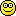191 / 9999 * 7 + 191 * 7SketchyCornwall UKRegistered  06/11/2004 Points  196017th July, 2009 at 02:18:21 - Big thanks to everyone who helped - it's much appreciatedI originally started out trying to solve this using trigonometry, as a number of you have suggested. With hindsight, I can see at least part of where I was going wrong: Finding the difference between the two lines - I just tried to subtract one from the other, instead of using the proper formula. As an example of why this didn't work, Angle_A = 45, Angle_B = 315. If you subtract one from the other, you get either 270 or -270. The actual difference though, is 90 degrees. If I'd remembered that then I probably would have been okay, apart from maybe having problems positive/negative angles somewhere down the line. Anyway, I eventually got it to work using just vectors, so I can't be bothered to try and do it again with trig.   n/a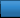AdvertisementWorth A Click